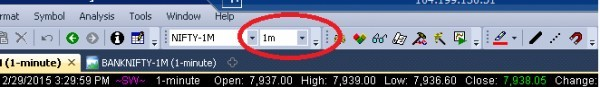# The following Amibroker afl is not working

Sir,

The following code i got from web.

I want to use this for exploration.

Also, one more query.

I am using exploration and getting results for some formulas.

But they are always giving resutls for daily values.

I want to use the code for checking 1 min, 5 min, 1 hour etc time frame what to add in the code?

(for example, I want to get values for 10 EMA of price. I am always getting only 10day EMA… how to get values if i need 10 period values for other time frames.)

/Volume Scan report for Amibroker (AFL)
//This afl gives SCAN REPORT of current script Volume greater then last 5 days Volume.
//Shows if intraday volume is greater than average volume of last 5 days (customizable) with moving average //line.

Plot(V,“Volume”,ParamColor(“Color”,colorBlueGrey),styleHistogram|styleThick);
Avgvol=0;
if (Interval()<inDaily) {
n = round(36006.75/Interval()); //number of bars in the day
d = Param(“Lookback days”,5,1,30,1); //averaging period
barnum = BarsSince(Day()!=Ref(Day(),-1)); //bar number from start of the day
for (i=0; i<n; i++) {
VarSet(“vol”+i,IIf(i==barnum,V,0));
}
for (i=0; i<n; i++) {
Avgvol=IIf(barnum==i,Sum(VarGet(“vol”+i),n
d)/d,Avgvol);
}
Plot(Avgvol,“Average Volume”,colorWhite,styleLine);
}
/*
for( i=0; i <n; i++ ) AddColumn(VarGet(“vol”+i),“vol”+i,1.0);
Filter=1;
*/

The above code is moving average on volume bars, and it is plotting check the below code

```Plot(V,"Volume",ParamColor("Color",colorBlueGrey),styleHistogram|styleThick);
Avgvol=0;
if (Interval()<inDaily) {
n = round(3600*6.75/Interval());  //number of bars in the day
d = Param("Lookback days",5,1,30,1); //averaging period
barnum = BarsSince(Day()!=Ref(Day(),-1)); //bar number from start of the day
for (i=0; i<n; i++) {
VarSet("vol"+i,IIf(i==barnum,V,0));
}
for (i=0; i<n; i++) {
Avgvol=IIf(barnum==i,Sum(VarGet("vol"+i),n*d)/d,Avgvol);
}
Plot(Avgvol,"Average Volume",colorWhite,styleLine);
}```

and if you want to plot in different time frame you have to set that  as shown in below screenshotThank you sir.
In chart the code is working.
I also want to check in explorer.
In explorer it is showing error. Please check.
Thanks